# MathBoolean

Library of Boolean mathematical functions as input/output blocks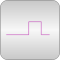# Package Contents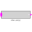MultiSwitch Set Boolean expression that is associated with the first active input signal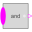And Logical 'and': y = u and u and ... and u[nu]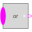Or Logical 'or': y = u or u or ... or u[nu]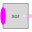Xor Logical 'xor': y = oneTrue(u) (y is true, if exactly one element of u is true, otherwise it is false)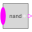Nand Logical 'nand': y = not ( u and u and ... and u[nu] )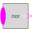Nor Logical 'nor': y = not ( u or u or ... or u[nu] )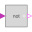Not Logical 'not': y = not u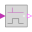RisingEdge Output y is true, if the input u has a rising edge, otherwise it is false (y = edge(u))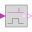FallingEdge Output y is true, if the input u has a falling edge, otherwise it is false (y = edge(not u))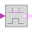ChangingEdge Output y is true, if the input u has either a rising or a falling edge and otherwise it is false (y=change(u))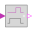OnDelay Delay a rising edge of the input, but do not delay a falling edge.

# Information

This information is part of the Modelica Standard Library maintained by the Modelica Association.

This package contains basic mathematical operations on Boolean signals.

The new features are:

• If useful, blocks may have an arbitrary number of inputs (e.g., "And" block with 2,3,4,... Boolean inputs). This is based on the "connectorSizing" annotation which allows a tool to conveniently handle vectors of connectors.
• The blocks are smaller in size, so that the diagram area is better utilized for trivial blocks such as "And" or "Or".